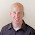# How to Teach Addition Without Using Fingers

How many of your students use their fingers to add numbers? I have some basic tips that will guide your students in the right direction to master addition skills and will not use their fingers. Students should master each step before moving on to the next step. The goal is to help students see numbers in a different way which will help them think about the numbers instead of counting on their fingers.
Here are the steps that will help students become proficient!

First, teach the numbers that make 10. For example: 1+9, 2+8, 3+7, 4+6, and 5+5. Students should be able to recognize these pairs of numbers instantly. This will help them later on too. If students know that 3+7=10, then later on they would know that 30+70=100. Plus, our place value system is based on tens. Students need to proficiently know these pairs that make 10. If you need extra practice, try this pack of making 10.
Here is a FREE game to learn the combinations or pairs of 10. This is a great game to help memorize the pairs. Just click the picture on the left to download this free game.
Next, teach adding a 10 to a number. For example; 3+10. This should be done with no thinking. Counting by 10's from any number is essential! They should NOT be counting 10 more on their fingers. This needs to be automatic. They should be able to count by 10's going forward and backwards from any number. Students should also be proficient at counting 1 more and 1 less from any number. Here is a FREE resource to help with adding and subtracting by 1's and 10's.Then, teach adding a 9 to a number. For example: 3+9. If they know how to add 10, they just subtract 1 less. This will help exercise the brain moving forward and backwards on a number line. Most students need help seeing the 9 in the equation. Sometimes they sit and stare at the addition fact with a blank stare until I say, "I see a 9." Then right away they know what to do. It's training the brain to see the 9 and connecting it with a trick they know. If you know how to add 10, then you can add 9 with ease. This is helpful when subtracting too. Students really need to be proficient in counting forward and backwards on a number line with simple arithmetic like 1 more or 1 less.

After, teach the doubles. For example: 5+5 or 6+6. Students should know their doubles without thinking. They should not be adding on their fingers a double. These need to be memorized. Doubles come in handy when thinking about other addition facts. Doubles also help when learning multiplication. Know those doubles! They should also recognize the subtraction fact family of a double too. If they know that 6+6=12, then they should begin to recognize that 12-6=6. Students can do a lot when they know their doubles.

After you teach the doubles, you can work on doubles +1. For example: 6+7. Some people like to call these numbers, neighbors, because they are neighbors or next door to each other on the number line. For this addition fact, you can see 6 and 7 are neighbors on the number line. If you see a neighbor, you take the smallest number, double it, and add 1 more. As students become proficient, they will begin learning tricks and seeing numbers differently. Their brain learns to look for combinations of numbers and connecting it with what they know.

Finally, students should be proficient in adding 2 more or 3 more to a number. They should already know how to add 1 more with ease. Adding another 1 is simple.

Once these steps are masters, students will be thinking in their heads combinations of numbers, looking for pairs that make 10 or a double, and begin using a strategy other than their fingers.

When they get to a fact like 4+7, they may think, "I know that 3+7 is 10, therefore 4+7 must be 11." or they may think, "I know that 4+4 is 8, therefore 3 more make 11." Or they may think, "I know that 4+6=10, then 1 more would make 11." These 3 examples use strategies other than fingers.

The best way to learn these strategies is to practice, practice, and practice. We can't be good at something if we don't practice. Right?

Teaching to take numbers apart like using Number Bonds and connecting them with what you know is very helpful. I found a YouTube video that explains Number Bonds pretty well.  Click here to watch a video about Number Bonds.

Here are some fun resources that will help master addition facts and to practice the strategies listed above.

Here are some more ideas to use when teaching to master facts:

See you soon,

I also offer all these products at a discount right here on my blog. Just click the picture on the left to find the resource that is just right for you and your students.
2.OA.2

1.2.3.4.